# Brief descriptions of content

Scroll down the page to view the modules listed with their descriptions or click on the math level listed in the table below to go directly to the math level of interest.

 Level 1 Math : Level 3 Math: Pre-algebra; arithmetic; substitution into formulas; computation; simple geometry Algebra with functions; evaluating algebraic functions; solving equations; graphing Level 2 Math : Level 4 Math: Algebra with equations (not functions); solving equations; reading graphs; geometry Pre-calculus; algebraic and exponential functions; evaluation; graphing; geometry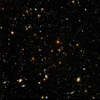KM#0: Age of the Universe—Introductory Module
Brief Description:  This study provides an introduction to the format for the Kéyah Math studies by example. The age of the Universe is computed illustrating the structure of a module and use of the applets.  Mathematical Content:  Basic algebra, linear equations, graphs

LEVEL 1 MODULE: Pre-algebra; arithmetic; substitution into formulas; computation; simple geometry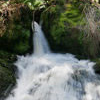KM#1: StreamFlow for the Animas River (Level 1)
Brief Description:   This study explores a very simplified method for predicting streamflow, or the amount of water that flows through a stream. After an introduction, the area of its watershed is computed. Then, given the average amount of precipitation, the volume of water contributed to the watershed is determined. This information is used to find the amount of water that goes into the stream, or the annual streamflow.  Mathematical Content: Area, Volume, Unit Conversion

LEVEL 2 MODULES: Algebra with equations (not functions); solving equations; reading graphs; geometry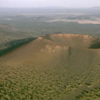KM#2: Sunset Crater (Level 2)

Brief Description:  This module is a study of ejecta from Sunset Crater, just north of Flagstaff Arizona. The velocity of bombs ejected from the crater during its eruption is determined using equations given to students.  Mathematical Content:  Basic algebra; solving equationsKM#3: Snow Melt and Stream Flow for the Animas River(Level 2)
Brief Description:   This study explores a simple way to predict streamflow, or the amount of water that flows through a stream. After an introduction, there are 3 parts, predicting temperature, precipitation (rain and snow), then the volume of water over the watershed is computed and used to predict streamflow. Then, given the average amount of precipitation, the volume of water contributed to the watershed is determined. This information is used to find the amount of water that goes into the stream, or the annual streamflow. Mathematical Content: Area, Volume, Unit Conversion, linear equations.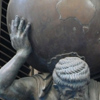KM#4: The Size of the Earth (Level 2)
Brief Description:  The goal of this study is to learn how Eratosthenes estimated the circumference of the earth.  After explaining the geometry used by Eratosthenes to estimate the circumference of the Earth, students use this information to compute the radius and volume.   Mathematical Content: Geometry (radius and circumference of a circle; volume of a sphere); interior angles.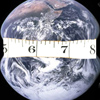KM #5: The Size of the Earth—Estimation in Arizona (Level 2)
Brief Description:  This study uses the method of Eratosthenes with local information from Arizona to estimate the circumference of the earth.  This estimate is then used to compute the Earth’s radius and volume. Mathematical Content: Geometry (radius and circumference of a circle; volume of a sphere); interior angles.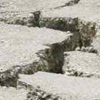KM#6: Location of the Epicenter of an Earthquake (Level 2)
Brief Description: This study examines the speed of P and S waves emitted from an earthquake, Equations relating distance traveled and speed are written, then the equations are used to find the epicenter as the intersection of three circles, each centered at a seismic station.  Mathematical Content: Basic algebra; equation of a line; radius of a circle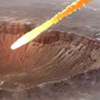KM#7: Impact Processes - Meteor Crater (Level 2)
Brief Description:  This study examines Meteor Crater, Arizona, and the size of the meteorite that formed it. After an introduction to impact processes, students are guided through a sequence of steps that involve relatively simple formulas from physics to find the size of the meteorite. Specifically: a formula relating kinetic energy and diameter of the crater is used to find kinetic energy released on impact; a formula relating KE and mass is used to find the mass of the meteorite; a formula relating mass density and volume is used to find the volume of the meteorite; and finally, a formula relating spherical volume to radius is used to find the diameter of the meteorite.  Mathematical Content:  Basic algebra, solving simple equations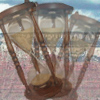KM#8: Age of the Earth (Level 2+)
Brief Description: This study explores methods for dating rocks using radioactive decay to find the age of rock from the San Juan Mountains and the age of the Earth. A basic decay equation for the  Rubidium-Strontium isotope system, is given and applied to date rock samples from Electra Lake, Southwest Colorado.  Then this equation is then used to date a meteorite containing rubidium and strontium to estimate the age of the Earth.  Mathematical Content: Basic algebra; exponential equations; logarithms.

LEVEL 3 MODULES: Algebra with functions; evaluating algebraic functions; solving equations; graphingKM#9: Mass and Density of the Earth (Level 3)

Brief Description:  This study is a follow-up to KM#4 or 5. It uses estimates of the radius and volume of the Earth to compute its mass and density .  Methods of Newton using the Law of Gravitational Attraction and density equations are used.  Mathematical Content: Geometry (radius and circumference of a circle; volume of a sphere); interior angles; Newton's Law of gravitational attraction; formulas for density and force; solving equations involving kinetic energy, mass and volume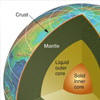KM#10: Layers of the Earth (Level 3)
Brief Description: This study explores using graphs of travel times of seismic waves to discover a layering structure of the Earth. After an introduction, and using results for the diameter of the earth found in module #4 or #5, the student is given information about the travel time of two seismic waves to a station a known distance from the epicenter of an earthquake.  The student computes the velocity of the first seismic wave and the distance the second wave travels.  From this information it is now possible to deduce the path of the second wave and conclude that the earth is layered.  The depth of this layer and the radius of the inner barrier are also computed.  Mathematical Content: Basic algebra (distance, rate and time); geometry; Snell’s lawKM#11: Size, Mass and Density of the Earth  (Level 3)
Brief Description:  The goal of this study is to learn how Eratosthenes estimated the circumference of the earth.  After explaining the geometry used by Eratosthenes to estimate the circumference of the Earth, students use this information to compute the radius and volume.  The students then apply the same method to local Arizona data and derive another estimate for the circumference and volume of the Earth.  Finally, this estimate is then used to compute the Earth’s density.  Mathematical Content: Geometry (radius and circumference of a circle; volume of a sphere); interior angles; Newton's Law of gravitational attraction; formulas for density and force; solving equations

LEVEL 4 MODULES: Pre-calculus; algebraic and exponential functions; evaluation; graphing; geometryKM#12: Age of the Earth (Level 4)
Brief Description: This study explores methods for dating rocks using radioactive decay to find the age of rock from the San Juan Mountains and the age of the Earth. A  basic decay equation for the  Rubidium-Strontium isotope system, is derived and applied to date rock samples from Electra Lake, Southwest Colorado.  Then this equation is used to date a meteorite containing rubidium and strontium to estimate the age of the Earth.  Mathematical Content: Basic algebra; exponential equations; logarithms; linear regression.KM#13: Impact Processes: Meteor Crater (Level 4)
Brief Description:  This study examines Meteor Crater, Arizona, and the size of the meteorite that formed it. After an extensive introduction to impact processes, students are guided through a sequence of steps that involve formulas from physics to find the size of the meteorite. Specifically: a formula relating kinetic energy and diameter of the crater is found using power regression on real data. This is used to find kinetic energy released on impact; a formula relating KE and mass is used to find the mass of the meteorite; a formula relating mass density and volume is used to find the volume of the meteorite; and finally, a formula relating spherical volume to radius is used to find the diameter of the meteorite. Mathematical Content:  Algebra, power and linear equations, linear regression, solving equations involving kinetic energy, mass and volume.

This material is based upon work supported by the National Science Foundation under Grant GEO-0355224. Any opinions, findings, and conclusions or recommendations expressed in this material are those of the authors and do not necessarily reflect the views of the National Science Foundation.